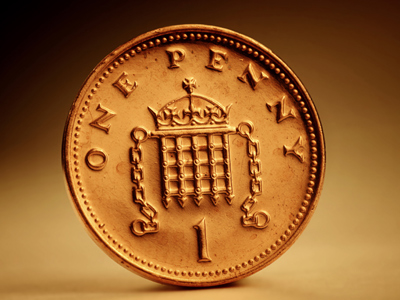There are 100 one penny pieces in a pound.

# Decimal Numbers 1

This Math quiz is called 'Decimal Numbers 1' and it has been written by teachers to help you if you are studying the subject at elementary school. Playing educational quizzes is an enjoyable way to learn if you are in the 3rd, 4th or 5th grade - aged 8 to 11.

It costs only \$12.50 per month to play this quiz and over 3,500 others that help you with your school work. You can subscribe on the page at Join Us

Decimal numbers are numbers that are not whole. 3, 7, 9 and 10 are whole numbers. 3.4, 7.1, 9.2 and 10.3 are decimal numbers. Can you spot the difference? The decimal numbers have a 'dot' which is called a decimal point. Adding and subtracting decimal numbers is just like doing it with whole numbers, for example 11 + 12 = 23 and 1.1 + 1.2 = 2.3. Decimal numbers can also be changed into the whole number they are closest to. We call this rounding up or down. For example, 4.7 would be rounded up to 5 but 4.4 would be rounded down to 4.

1.
Which is the largest?
2.9
3.4
4.3
0.8
All the other numbers have less than 4 units
2.
How is 0.8 changed to 0.2?
Subtract 0.6
Subtract 6
Just like 8 - 6 = 2
0.8 - 0.6 = 0.2
3.
What is £19.42 rounded to the nearest £?
£18
£19
£19.50
£20
We round down numbers less than .5 and we round up numbers equal to or higher than .5
4.
What is the figure 7 worth in 1.7?
7 units
7 hundredths
7 tenths
7 tens
A number 7 in the tenths column means 710
5.
Which is the decimal fraction equivalent to thirty two and five tenths?
3.25
32.5
0.325
325
Whole numbers are on the left of the decimal point
6.
What is 423cm in meters?
423m
42.3m
4.23m
0.423m
There are 100cm in a meter. Therefore 423cm is 4m and 23cm or 4.23m
7.
What is 345p in £s?
£3.45
£345
£0.345
£34.5
There are 100 pence in £1 so we divide 345 by 100 which gives 3.45 or £3 and 45p
8.
Which is the smallest?
1.7
0.9
2.1
0.3
310 is less than 910
9.
How is 4.3 changed to 4.7?
Subtract 0.4
Divide by 0.4
Just like 43 + 4 = 47,
4.3 + 0.4 = 4.7
10.
What does the digit 4 represent in 3.4?
4 tenths
4 tens
4 units
4 hundredths
Tenths are the column after the decimal point
Author:  Amanda Swift